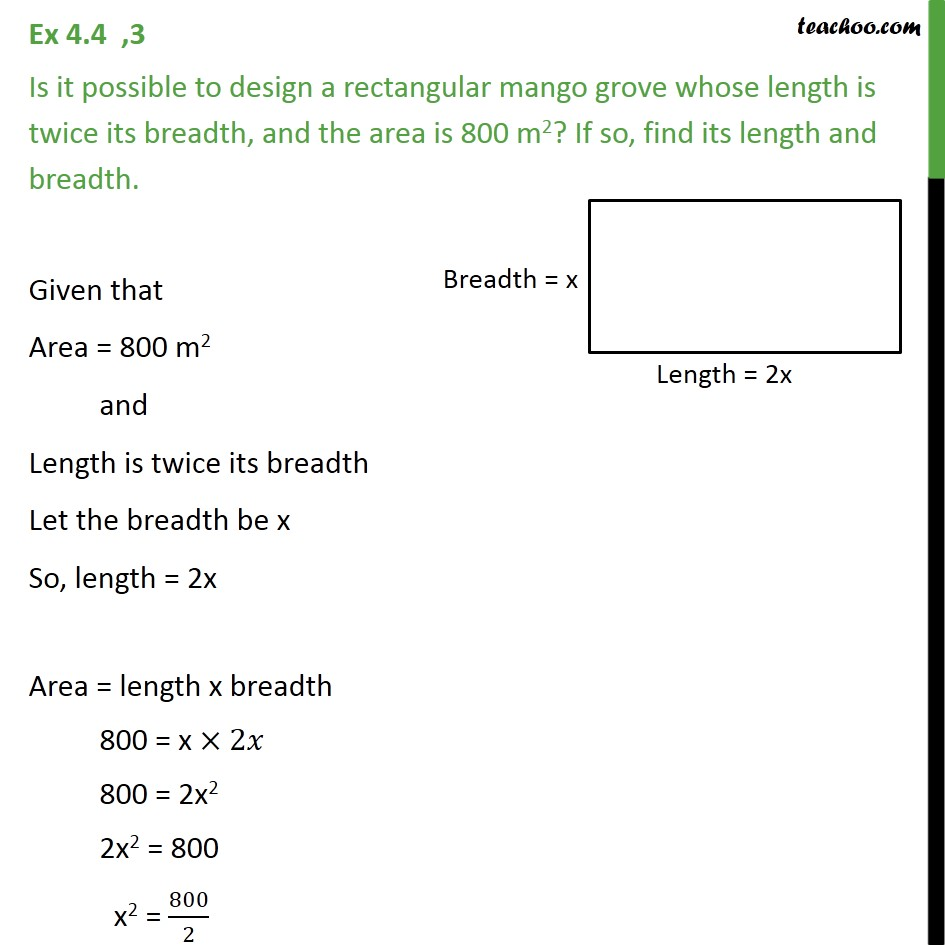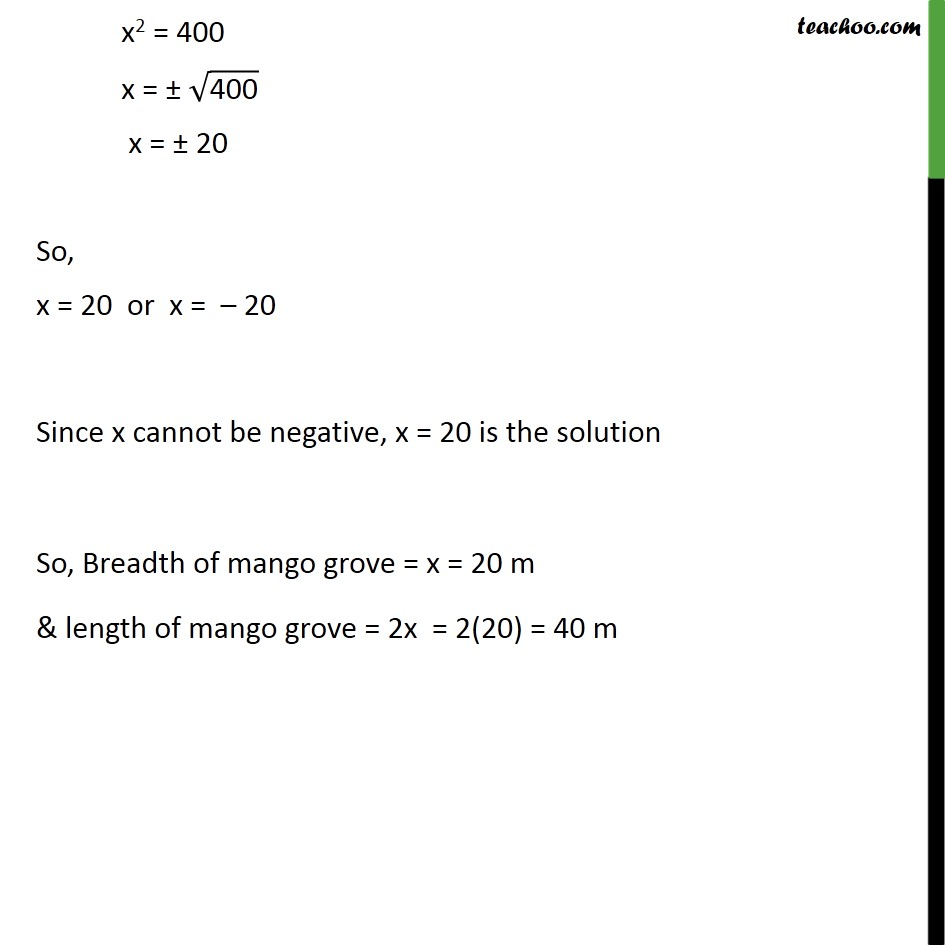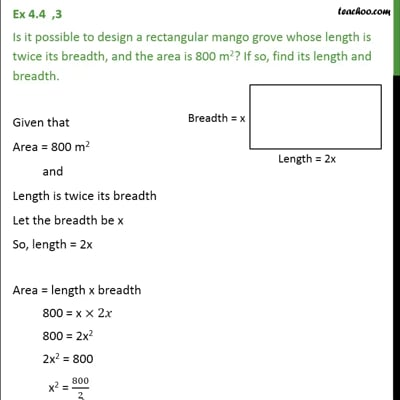Ex 4.4

Chapter 4 Class 10 Quadratic Equations (Term 2)
Serial order wiseThis video is only available for Teachoo black users

### Transcript

Ex 4.4 ,3 Is it possible to design a rectangular mango grove whose length is twice its breadth, and the area is 800 m2? If so, find its length and breadth. Given that Area = 800 m2 and Length is twice its breadth Let the breadth be x So, length = 2x Area = length x breadth 800 = x 2 800 = 2x2 2x2 = 800 x2 = 800/2 x2 = 400 x = ("400" ) x = 20 So, x = 20 or x = 20 Since x cannot be negative, x = 20 is the solution So, Breadth of mango grove = x = 20 m & length of mango grove = 2x = 2(20) = 40 m